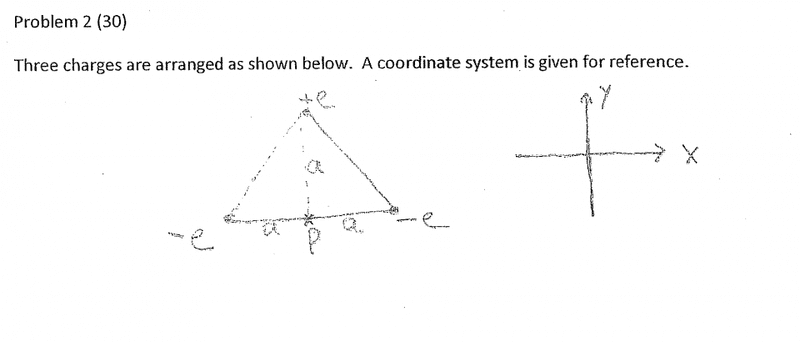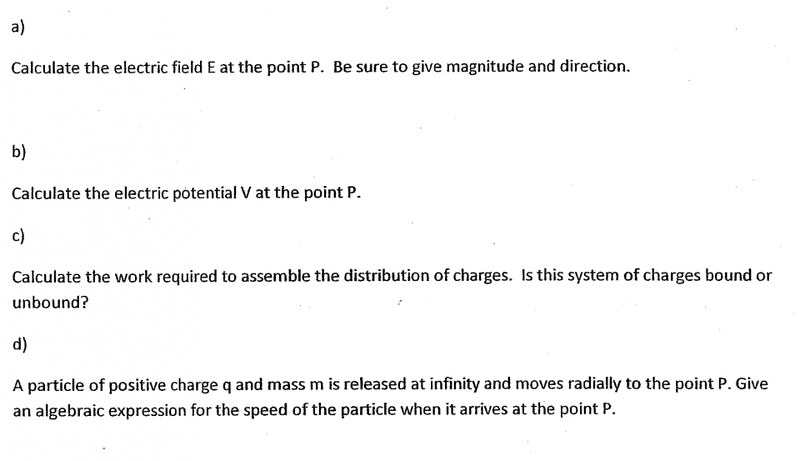# Electric Field, Potential, and Work at a Point

## Homework StatementE = kq/r2
V = -∫ E ⋅ ds
W = -Vq

## The Attempt at a Solution

a) I just summed up the electric fields: +ek/a2 +ek/a2 -ek/a2 so I get +ek/a2 as a result. Not sure if this is correct since -e has a y component so I was thinking to use sin but not sure which angle to use.

b) i would just plug in the electric field from part a and plug in a as the distance, so V = -Ea

c) W = -Vq, get the potential from b and for q get the sum of charges. Not sure if that's a correct approach.

d) I'm not sure what equation to use for this.

a) I just summed up the electric fields: +ek/a2 +ek/a2 -ek/a2 so I get +ek/a2 as a result. Not sure if this is correct since -e has a y component so I was thinking to use sin but not sure which angle to use.

b) i would just plug in the electric field from part a and plug in a as the distance, so V = -Ea

c) W = -Vq, get the potential from b and for q get the sum of charges. Not sure if that's a correct approach.

d) I'm not sure what equation to use for this.

a. electric field intensity is a vector - so you must put in the direction of the fields at P due to charges -use the symmetry of the figure;
as your charges -e are equidistant from the point p. so get a correct value of the field at P

b. if you know the field you can also calculate the potential due to the charges -these are scalars therefore it will be added
c. what is the condition that charges will be bound or fly apart?
d. calculate the work done in bringing an unit positive charge from infinity to the point p -is it related to potential at that point.?
can work done by the field related to speed of the particle ?
some questions are to develop one'concept about charges and fields.

•